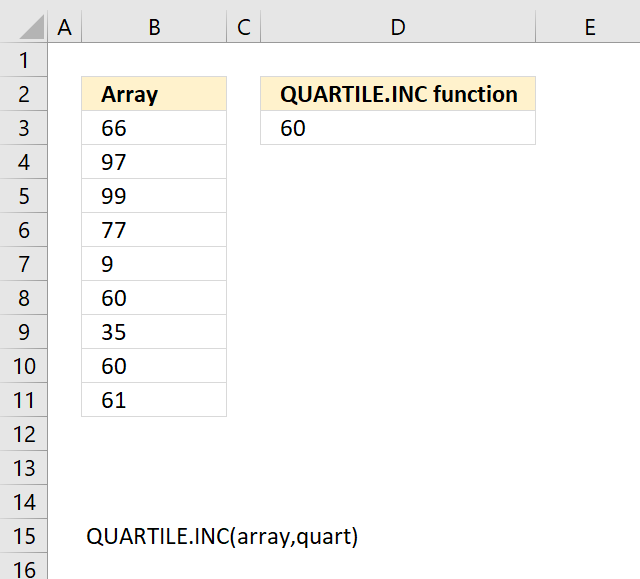Author: Oscar Cronquist Article last updated on April 20, 2018The QUARTILE.INC function returns the quartile of a data set, based on percentile values from 0..1, inclusive.

Use the Quartiles.inc function to divide numerical values into groups.

Formula in cell E3:

=QUARTILE.INC(B3:B11,1)

### Excel Function Syntax

QUARTILE.INC(array, quart)

### Arguments

 array Required.The cell values for which you want to calculate the quartile value. quart Required. Indicates which value to return.
Quart parameters
0 Minimum value.
1 First quartile (25th percentile).
2 Median quartile (50th percentile).
3 Third quartile (75th percentile).
4 Maximum value.